# ISEE Lower Level Math : How to find the perimeter of a rectangle

## Example Questions

1 2 3 4 6 Next →

### Example Question #141 : Geometry

A rectangle measuresinches along its short side, andinches along its long side.  What is the perimeter of the rectangle?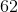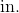Explanation:

To find the perimeter of any object, simply add the length of each side together.  In the case of the rectangle, there are two short sides, and two long sides, so you can also multiply the short side by two, the long side by two, and add the sums.  The best answer is: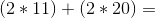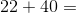inches

### Example Question #142 : Geometry

Find the perimeter of a rectangle with a length of 16in and a width that is half the length.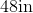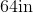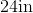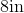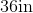Explanation:

To find the perimeter of a rectangle, we will use the following formula: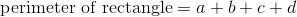Where a, b, c, and d are the lengths of the sides of the rectangle.

Now, we know the length of the rectangle is 16in.  Because it is a rectangle, the opposite side is also 16in.

We know the width is half the length.  Therefore, the width is 8in. Because it is a rectangle, the opposite side is also 8in.

Knowing this, we can substitute into the formula.  We get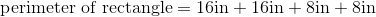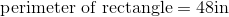### Example Question #143 : Geometry

Find the perimeter of a rectangle with a width of 7cm and a length that is two times the width.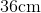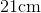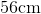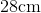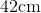Explanation:

To find the perimeter of a rectangle, we will use the following formula: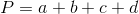Where a, b, c, and d are the lengths of the sides of the rectangle.

Now, we know the width is 7cm.  Because it is a rectangle, the opposite side is also 7cm.

We know the length is two times the width.  Therefore, the length is 14cm.  Because it is a rectangle, the opposite side is also 14cm.

Knowing this, we can substitute into the formula.  We get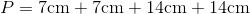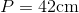### Example Question #144 : Geometry

Find the perimeter of the following rectangle: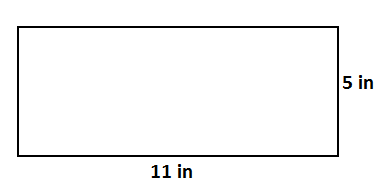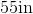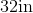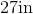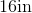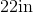Explanation:

To find the perimeter of a rectangle, we will use the following formula:Where a, b, c, and d are the lengths of the sides of the rectangle.

Now, given the rectanglewe can see the length is 11in.  Because it is a rectangle, the opposite side is also 11in.

We can see the width is 5in.  Because it is a rectangle, the opposite side is also 5in.

Knowing this, we can substitute into the formula.  We get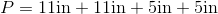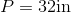### Example Question #145 : Geometry

Find the perimeter of a rectangle with a length of 15in and a width that is a third of the length.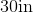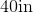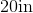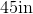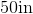Explanation:

To find the perimeter of a rectangle, we will use the following formula:Where a, b, c, and d are the lengths of the sides of the rectangle.

Now, we know the length of the rectangle is 15in.  Because it is a rectangle, the opposite side is also 15in.

We know the width of the rectangle is a third of the length.  Therefore, the width of the rectangle is 5in.  Because it is a rectangle, the opposite side is also 5in.

So, we get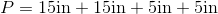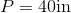1 2 3 4 6 Next →